Constructing a triangle using SAS congruency criteria

Practical Geometry
Serial order wise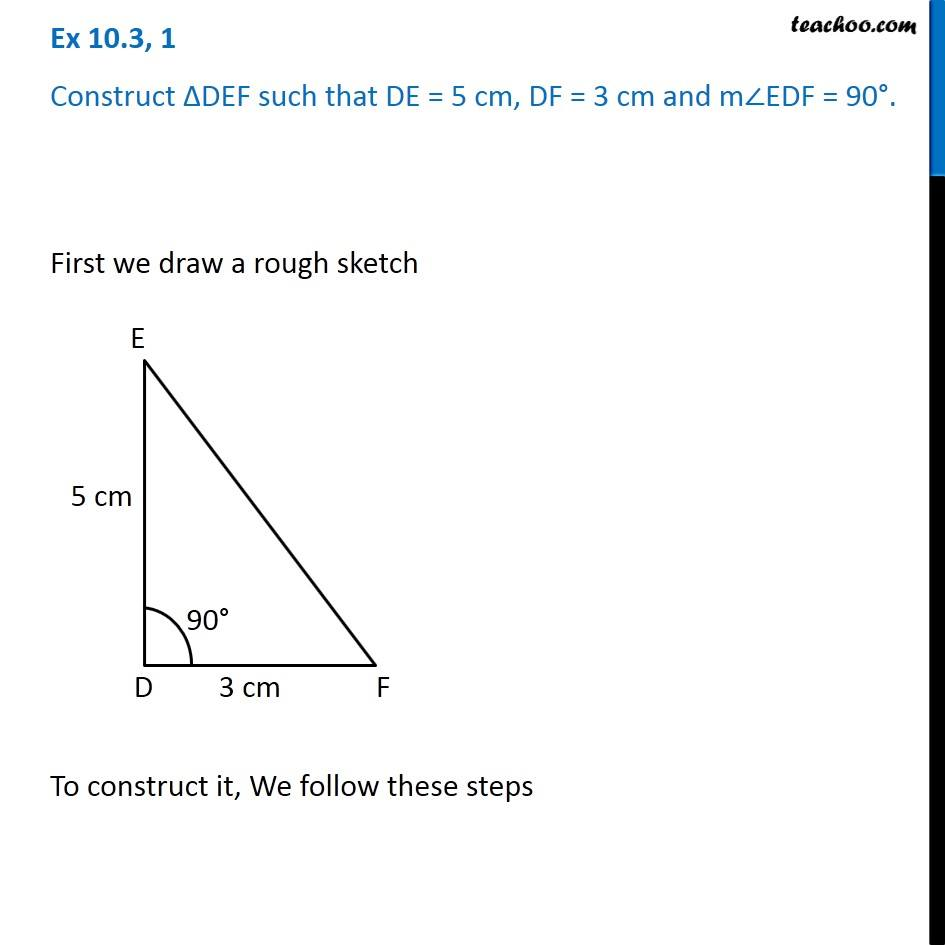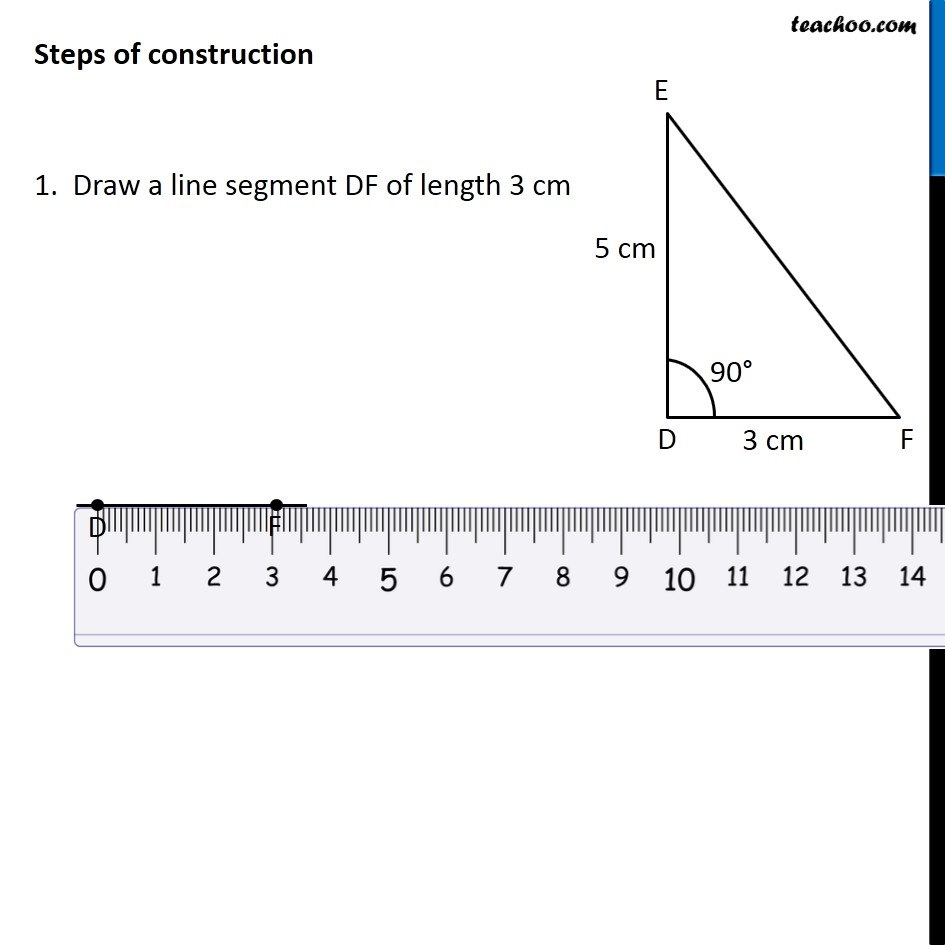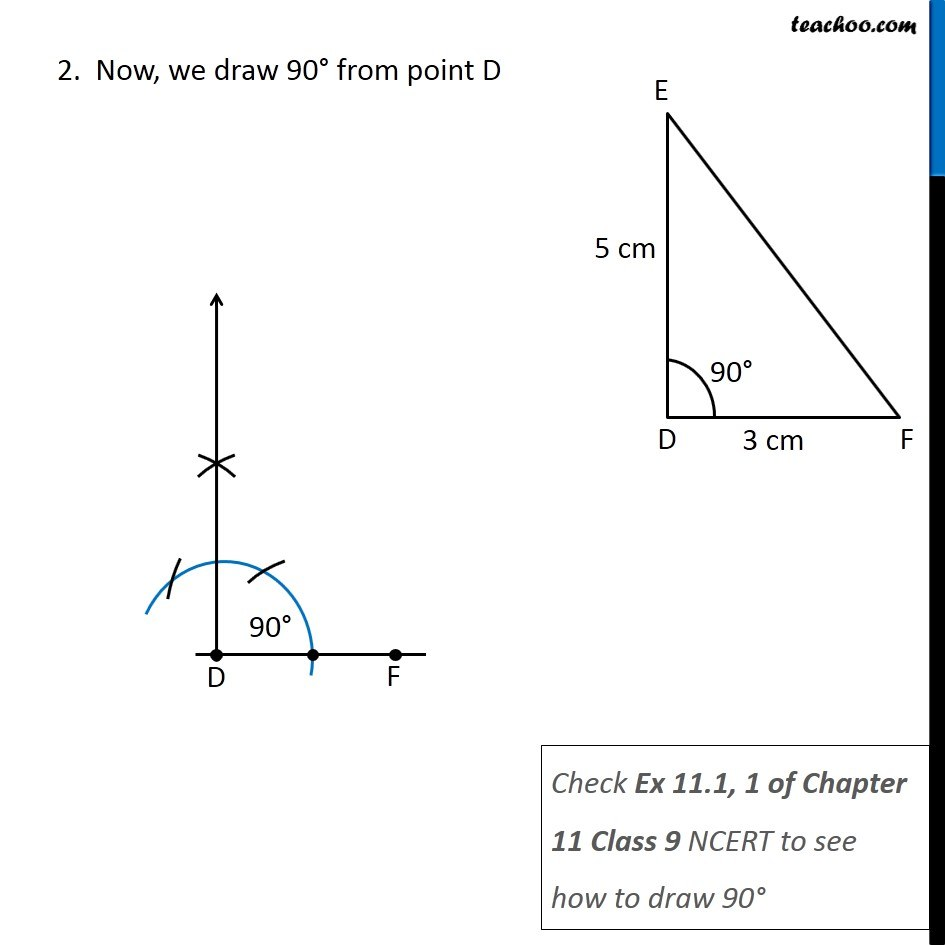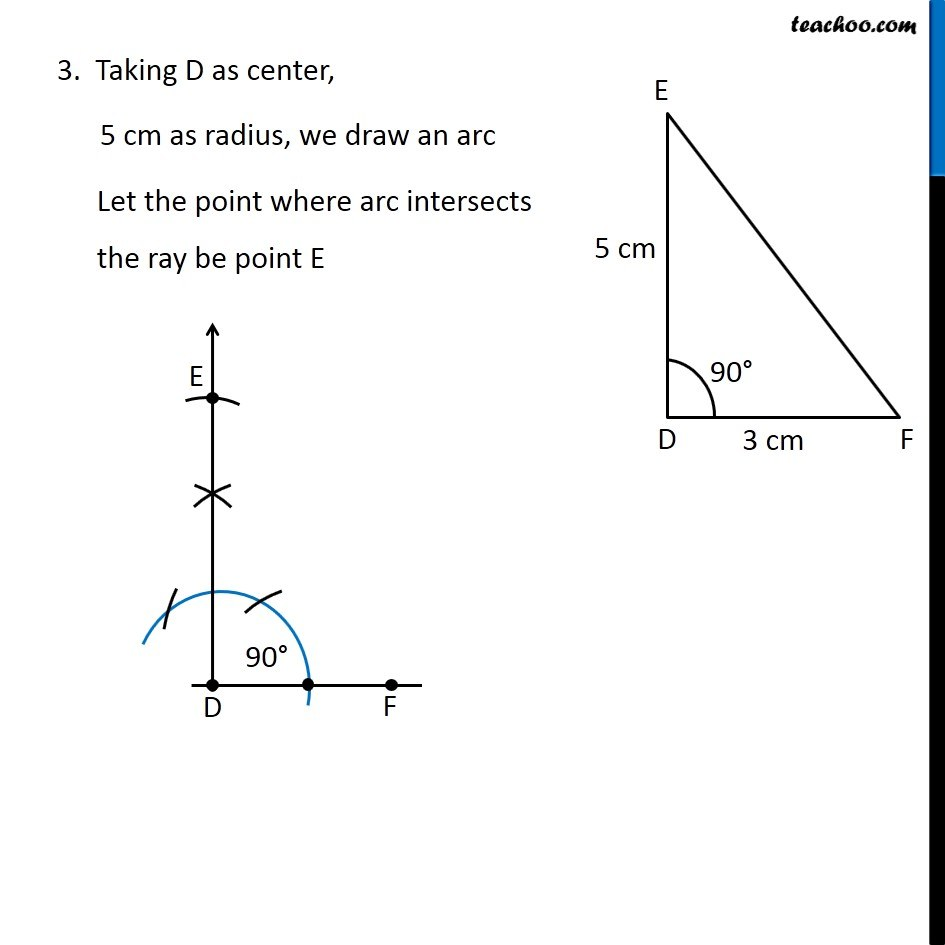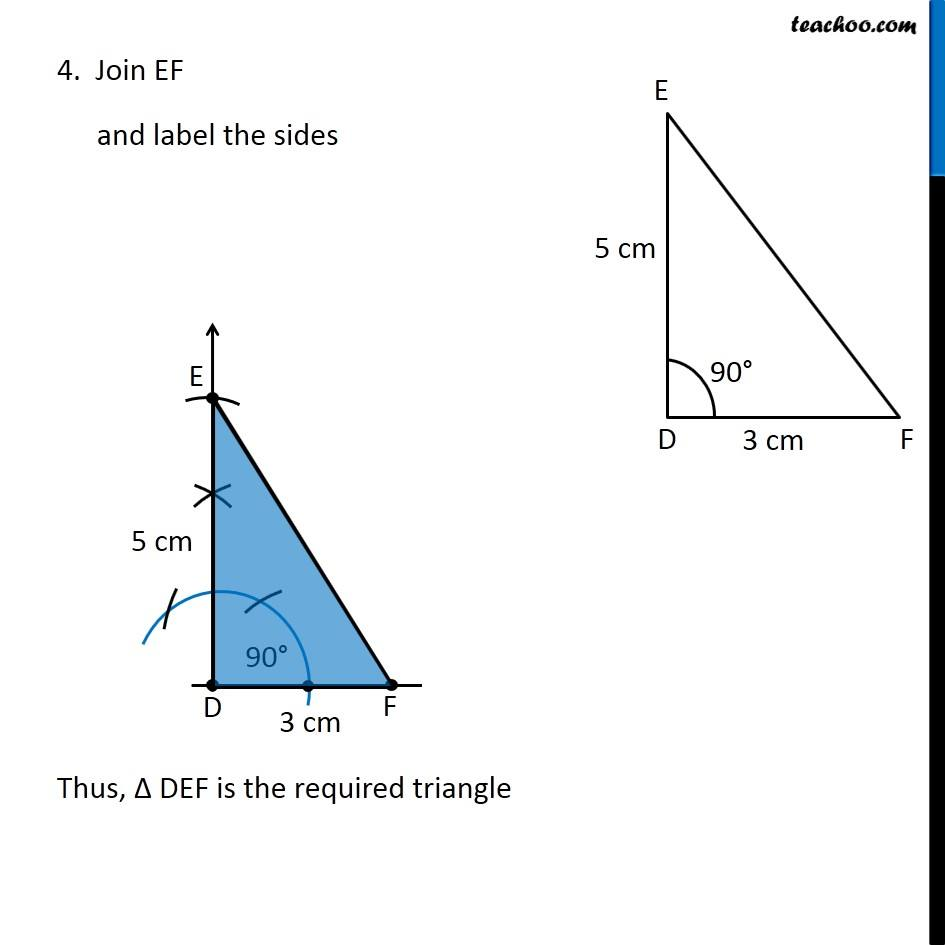Learn in your speed, with individual attention - Teachoo Maths 1-on-1 Class

### Transcript

Question 1 Construct ∆DEF such that DE = 5 cm, DF = 3 cm and m∠EDF = 90°. First we draw a rough sketch To construct it, We follow these steps Steps of construction 1. Draw a line segment DF of length 3 cm 2. Now, we draw 90° from point D Check Ex 11.1, 1 of Chapter 11 Class 9 NCERT to see how to draw 90° 3. Taking D as center, 5 cm as radius, we draw an arc Let the point where arc intersects the ray be point E 4. Join EF and label the sides Thus, Δ DEF is the required triangle